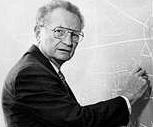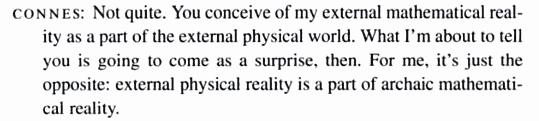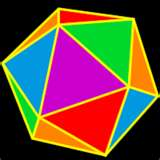# Mathematics is not (only) a language

Posted by: Gary Ernest Davis on: January 9, 2010

Every so often someone will assert that mathematics is a language.

What Â concerns me about the statement “mathematics is a a language” Â is what I am not hearing directly: “mathematics is ONLY a language” Â which I worry is implicit.

For example in an interview, Paul Samuelson, Â said:Well, my belief is that nothing that can be expressed by mathematics cannot be expressed by careful use of literary words. All the mathematics itself originally grew out of axioms — assumptions stated in words — and [it reminds me of] the only speech that the great Willard Gibbs, at Yale, ever gave at a faculty meeting, when there was a debate between studying languages and mathematics. He said four words: “mathematics IS a language.” And that’s on the frontispiece of my Foundations of Economic Analysis, but I’ve written somewhere that he should have cut it down to three words: “Mathematics is language.” It’s just a very compact form of language.

So there we have it from the winner of the 1970Â Sveriges Riksbank Prize in Economic Sciences in Memory of Alfred Nobel himself: Mathematics is just a very compact from of language.

This view of mathematics – that it is just a language for expressing something about another topic of interest, such as physics, economics, chemistry, sociology – Â is common among users of applied mathematics For such people mathematics is not their major concern. Rightly so – their focus of attention is on physics, or chemistry, or economics. If they could avoid mathematics altogether they probably would.

But what about people who are mathematicians: are they really just a bunch of linguists, getting together to discuss the language of mathematics? Wouldn’t you think that the people whose life is mathematics might know if mathematics is just a language?

My own view is that mathematics is a science – it is Â a science of number, space, pattern and arrangement.

If this view has any validity then mathematics ought to have well established facts. Does it? You bet it does, and one of the great grand-daddy facts of mathematics is the prime number theorem.

The prime number theorem is not “just language”. It is a deep fact about the way prime numbers are spaced out in the collection of whole numbers. It was discovered empirically by Gauss and Legendre, and proved to be true by Jacques Â Hadamard.

The prime number theorem asserts that the$n^{th}$ prime number is approximately equal to$n \log (n)$, with the relative error of this approximation approaching 0 as$n$Â  increases without bound. Â Here$\log (n) =\int_1^n\frac{1}{x}dx$ is the natural logarithm of$n$: Â the area under the curve$y=\frac{1}{x}$ from$1 \textrm{ to } n$.

Alain Connes has asserted that we know such facts about mathematical reality better than we know any fact about physical reality. He writes:

The scientific life of mathematicians can be pictured as a trip inside the geography of the mathematical reality which they unveil gradually in their own private mental frame.” [A_view_of_mathematics]

Connes has even gone so far as to assert:Is this an isolated example, essentially the only real fact in mathematics. No, to the contrary, mathematics is full of mathematical facts.Here’s another simple, but beautiful fact discovered by Euler and overlooked by the ancient Greeks in their study of polyhedra. A polyhedron has a number of vertices (v), edges (e) and faces (f). Euler discovered, and proved, that v-e+f=2. Â Is this just a language statement. No: it is a provable, but non-obvious, fact about plane graphs which applies to the graphs of Â polyhedra.

Dave Richerson has a beautiful account of this lovely finding of Euler in his book Euler’s Gem. If you still think that mathematics is (only) a language, do yourself, and me, a favor by getting hold of this book and reading it with pleasure. It is a beautifully written book, that tells what the science of topology is about.

So mathematics is a subject with deep facts, and is not just a language for expressing facts about some other topic.

Where, then does the idea that mathematics is just a language come from? I have already said that users of mathematics in other fields often see mathematics this way. But where did they learn that? My view is that school mathematics by and large promotes this idea that mathematics is just a language, because school mathematics is, by and large, devoid of mathematical facts. School mathematics is, in the main, a collection of recipes and methods for doing … who knows what.

It’s time, in my view, for an infusion of facts and mathematical science into school mathematics. Â Then we might get kids loving mathematics, as we might if we read Dave Richerson’s lovely book.

### 10 Responses to "Mathematics is not (only) a language"That’s funny because I find that in school it’s just the opposite. Students think mathematics is nothing BUT facts. The only way to succeed is to learn all the facts, i.e. the answer to every possible sum, product, or in the case of abstract math like algebra and higher, the exact algorithm for every permutation of variable, constant, exponent, and coefficient combination. When I have been able to get students to understand the language aspect of mathematics, then the conceptual understanding began to take place.

I realize that the bulk of the above article deals with mathematics of a much higher level than I teach in middle school or taught in high school. However, if we are going to prepare future mathematicians who love the language and its manipulation of facts, how that leads to discoveries that open up new practical realities or just plain geeky cool appreciations of a new mathematical beauty, students need both the facts and the language. (Sorry for the long sentence) The problem is education is that unfortunately, many of our educators do not understand the language themselves.Hi Matt,

thanks so much for the thoughtful comment.

I think a lot depends on what we see as a fact.

“Knowing that” is different from “knowing why”.

Most school mathematics facts are of the “knowing that” variety – maybe somebody told us, or we read it somewhere, or we have to know it for a test.

Outside of school, mathematical facts are mostly of the “knowing why” variety – what reason is there that this is true?I think the reason has much to do with the emphasis on standardized testing. It’s much easier to assess “knowing that” over “knowing why”.

I agree with you that the science of math should not be forgotten, but neither should the communication aspect. After you’ve discovered why something is true, you also need to be able to explain why it is true to someone else. For this communication to happen, there just needs to be some common language between parties.

It just so happens that the “language of Mathematics” is the most commonly used language for talking about Mathematics, so that’s what the standardized tests use. Since the major tests all use it, that’s what educators are forced to teach. If the SAT math questions were written in a language like LISP, I’m pretty sure that’s what the curriculum would be teaching to instead.When a deep mathematical fact is well enough understood, someone comes up with a compact notation for it. Think for example of div, grad and curl and the messy equations they replace. And that notation makes it easier both to learn the fact and move beyond it. It’s because of this cycle that a problem like Pons Asinorum goes from being a challenging problem in the Middle Ages to a simple high school exercise.

Thus, the power of mathematics as a language lies in its ability to express profound ideas. Without the ideas, it’s nothing.Thak you for this post! I’m basically mathematically illiterate, but it brought back some cherished memories of a departed friend who used to talk about Euler’s theorem about polygons.

I can’t find an email address, so just to tell you I’ve linked to the post on a weekly “highlight” post I do, 4th down. Hope this is ok.As a linguist, I bristle at the implied “only.” Even if mathematics is a language, so what? It doesn’t logically follow that if mathematics is a language, then anything expressed mathematically can be expressed in another language at all, much less equally well. See, for example, the Sapir-Worf hypothesis: language shapes thoughts, and the language one speaks influences the way one’s mind works. Even if mathematics is a language, knowing and communicating in mathematical language creates a different way of knowing.

The belief that mathematics is “only” a language undervalues language.Ted, I feel your reply missed a point I was trying to make on several counts. I perhaps could have expressed things more clearly.

People who say mathematics is “just” a language or “only” a language are trying to say that mathematics has no subject matter – that it is merely a particular way of expressing something about something else. My point is that mathematics has intrinsic “aboutness”, some of which is regarded by some people as deeper and more realisitic knowledge than our knoweldge of the physical world.

The Sapir-Worf hypothesis has had to be significantly modified, and while it has some validity, it is nowhere near as universally valid as was initially proposed by Sapir and Worf.

I do not think anyone is undervaluing language. Language is language, and we would be less than human without it. But mathematics is not simply a language – a means of expression. It is more than that, as is physics, chemistry and biology. It is a subject about something (something that many well known linguists deny). That aboutness requires study and thought to appreciate.Mathematics is a language in the sense that it is a formal construction based on axioms and valid in its own. Saying this doesn’t imply that mathematics is not about something… as it is about numbers, space, patterns and such abstract categories, and those, of course, have their own “facts”, none of those deducted from physical reality, but from its own set of internal rules. I believe those who say mathematics is a language aren’t implying it is only a tool to be used by other subjects (we most bear in mind pure mathematics has no practical application), but rather referring to the way it is built.This question is like asking questions “Is science just a language ?”. Answer I would think for most of would be “NO”.

Similarly I think Mathematics came about as basic notations [Language] to explain certain things around us. But grew into way to express certain observed reality in logically consistent manner.

But has evolved in two different ways

a) Being used to describe observed reality [This is where it helps science more a language form]
b) Being used to derive what all could be reality [Pure Maths]. this where it is amazing. Exploring the expressions in maths further and further explains things around us which we can’t even observe. It defines the outer boundaries of truth as we know it.

That is where Mathematics is different from Science [It needs it to be observable].

Coming back to question is “Mathematics just a language”. Answer would be “NO” but is it a science answer again would be “NO”… It is Mathematics.

One questions which may come up is

“Is theory of relativity Maths or Science ??”First, mathematics isn’t a language at all. There is a kind of language attached to mathematics that makes it easier to work in mathematics and communicate mathematics, but that language isn’t strictly necessary. Plenty of beautiful mathematical work was accomplished (albeit perhaps with a good deal of extra mental effort) before that language was developed.

…mathematics… is a science of number, space, pattern and arrangement.

I’m curious — is this how you would define “mathematics”? (I’ve been looking for a fitting definition of mathematics for a couple of months.) If so, might it not be better to amend it to “Mathematics is THE science of number, space, pattern and arrangement”? But in defining mathematics, I don’t know if it’s best to simply list some of the broad themes that mathematicians study. Is there a more concise and unifying statement that would capture the essence of what mathematics really is?

Sorry if that’s a bit tangential.